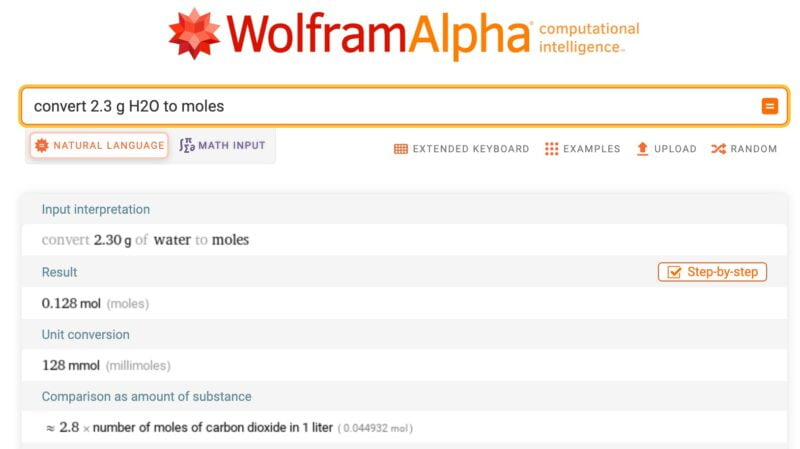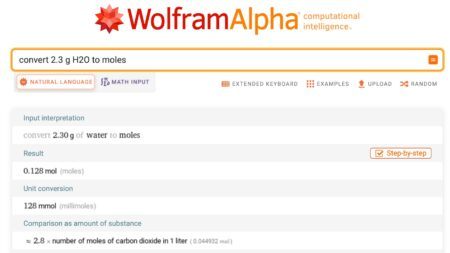This post highlights the potential impact of Wolfram|Alpha on the course curriculum in math and chemistry. It provides examples of how the tool can be used in chemistry, such as converting units, providing detailed chemistry information, comparing the chemistry of different elements or compounds, and solving chemical formulas. The author emphasizes the importance of exploring the possibilities of integrating Wolfram|Alpha into teaching and learning strategies.

So, perhaps we’re struggling a bit with the possibility of our course curriculum shifting a bit in math with the introduction of Wolfram|Alpha.   If you’re not struggling a bit, perhaps you haven’t played with it enough yet to really grasp the implications here.

Just so you know, whether they know it or not, the chemistry educators are going to have to think about this too.  Here are some examples:

Wolfram Alpha will convert units.

It will give you all the chemistry of an element, a compound, or something more complicated (make sure to click on “more”).

It will compare the chemistry of several elements or compounds.

It will solve a chemical formula for a specific variable (make sure to click on “show steps”).

Wolfram Alpha will do chemical conversions for you or do formula calculations.If there’s a chemist in your circle of friends, perhaps it’s time they got to join in the fun!